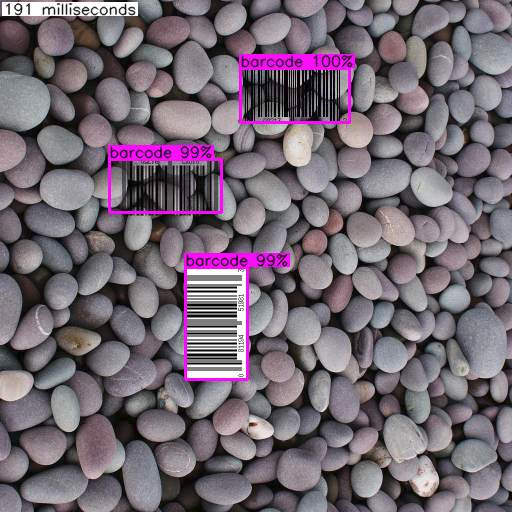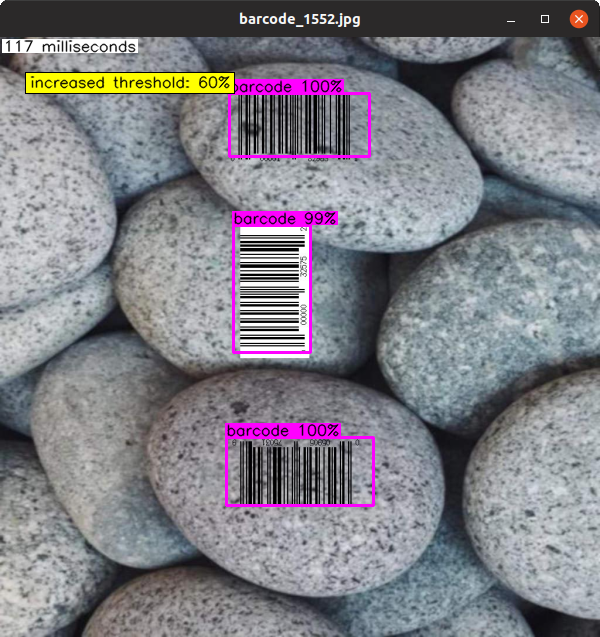../
This is post #6 of a 6-part series on Darknet. If you've not yet read the summary, I suggest you start there.

## Summary

Assuming you've followed the previous few posts, you should have a neural network trained with Darknet that works reasonably well to find barcodes-on-stones. The problem is every time you want to run an image through the neural network, this requires the darknet tool, which takes a non-trivial amount of time to start, and it doesn't integrate well into an application.

The good news is darknet has a C API which can be called upon. This post will explain how to access darknet from C and C++.

## C API

As part of the build steps described in this post, you should have a file named libdarknet.so in /usr/local/lib, and darknet.h in /usr/local/include. However, since darknet's C API is undocumented, it can be difficult to initially understand, and there isn't much example code.

The key is to find the function test_detector() in darknet's detector.c. In this function, you'll find the following calls:

• parse_network_cfg_custom()
• resize_image()
• network_predict()
• get_network_boxes()
• draw_detections_v3()

Most of these calls map to the C API available in libdarknet.so. The hardest part to understand was the probability pointer for each object found. The probability pointer is actually an array of probabilities where the array size matches the number of classes in the network. This can be seen in draw_detections_v3() in image.c. That code is not easy to understand, and I had to walk through it in a debugger many times to finally understand what it is doing.

## C++ API

Which brings us to the C++ API that I wrote. I took what I learned after using darknet's C API, and created a C++ library called DarkHelp. I wrote it so I wouldn't have to deal with the complications of the original C API. DarkHelp is a C++ class, and there are only three things to call:

• DarkHelp's constructor which loads the .cfg, the .weights, and the .names file.
• DarkHelp::predict() to analyze an image and get back a vector of detection results. This can be called many times, with many different images to analyze.
• DarkHelp::annotate() which applies the detection results to the image. This can also be called as many times as necessary.

If you're looking for a C++ API -- or some additional code to understand how darknet's original C API works -- you can download DarkHelp from here.

You'll still need darknet built and installed since DarkHelp uses darknet.h and libdarknet.so. From your C++ application, include the DarkHelp header file, then make the appropriate calls depending on whether you're looking to get back an annotated image, or a vector of detected objects. An example:

#include <DarkHelp.hpp> int main() { const std::string config_file = "stone_barcodes_yolov3-tiny.cfg"; const std::string weights_file = "stone_barcodes_yolov3-tiny_final.weights"; const std::string names_file = "stone_barcodes.names"; // load the neural network (config, weights, and class names) DarkHelp darkhelp(config_file, weights_file, names_file); // run the neural network predictions on an image, and save the output darkhelp.predict("barcode_7.jpg"); cv::Mat image = darkhelp.annotate(); // use standard OpenCV calls to write the annotated image to disk cv::imwrite("output.jpg", image, {cv::IMWRITE_JPEG_QUALITY, 65}); return 0; }

The results from output.jpg generated by the code block above looks like this:While at times visually impressive, seeing annotated images is likely not the reason why people want to use a neural network within their C/C++ application.

Instead, you need to act on the information that the neural network has put together. There are two things to note about the call to darkhelp.predict("barcode_7.jpg") in the example above:

1. There is an overload that takes a OpenCV cv::Mat, so the image to analyze does not have to come from disk.
2. The method predict() returns a vector of result structures. These results contain information on various things like the bounding box and the probablity scores between 0.0 and 1.0 for each class in the network.

So instead of annotating images, you can iterate through the vector of results and determine exactly what you want to do about the objects the neural network has detected. For example:

for (int idx = 1; idx < argc; idx++) { const std::string filename = argv[idx]; std::cout << "getting predictions on " << filename << std::endl; const auto results = darkhelp.predict(filename); std::cout << "prediction took " << darkhelp.duration_string() << std::endl; for (const auto & detection : results) { std::cout << "-------------------------" << std::endl << " name: " << detection.name << std::endl << " rect: " << "x=" << detection.rect.x << " " << "y=" << detection.rect.y << " " << "w=" << detection.rect.width << " " << "h=" << detection.rect.height << std::endl << " class: " << detection.best_class << std::endl << " prob.: " << detection.best_probability << std::endl; } }

That same image above with the 3 barcodes in it looks like this when we iterates through the results returned by DarkHelp::predict():

getting predictions on barcode_7.jpg prediction took 138 milliseconds ------------------------- name: barcode 100% rect: x=476 y=138 w=225 h=104 class: 0 prob.: 0.999623 ------------------------- name: barcode 100% rect: x=378 y=527 w=117 h=238 class: 0 prob.: 0.999203 ------------------------- name: barcode 100% rect: x=211 y=320 w=248 h=102 class: 0 prob.: 0.99849

## Stone Barcodes

The previous few posts contain all of the source code and instructions required to rebuild the "stone barcode" examples I've been using. Combined with the DarkHelp library (MIT open source license) this means you can run the same samples and examples that I have been using. But in case you don't want to wait for your neural network to finish training, I'm also making the darknet configuration and weight files available here: 2019-08-25_DarkHelp_Stone_Barcodes.zip. (Beware, the download is 32 MiB in size.) This contains the sample .cpp file from this post, the darknet neural network files, and 10 sample "stone barcode" image files against which you can test.This concludes the 6-part blog post on darknet, neural networks, and DarkHelp. Let me know if it has helped!